NEET  >  Test: Calculations Involving Unit Cell Dimensions (NCERT)

# Test: Calculations Involving Unit Cell Dimensions (NCERT) - NEET

Test Description

## 5 Questions MCQ Test Chemistry Class 12 - Test: Calculations Involving Unit Cell Dimensions (NCERT)

Test: Calculations Involving Unit Cell Dimensions (NCERT) for NEET 2023 is part of Chemistry Class 12 preparation. The Test: Calculations Involving Unit Cell Dimensions (NCERT) questions and answers have been prepared according to the NEET exam syllabus.The Test: Calculations Involving Unit Cell Dimensions (NCERT) MCQs are made for NEET 2023 Exam. Find important definitions, questions, notes, meanings, examples, exercises, MCQs and online tests for Test: Calculations Involving Unit Cell Dimensions (NCERT) below.
Solutions of Test: Calculations Involving Unit Cell Dimensions (NCERT) questions in English are available as part of our Chemistry Class 12 for NEET & Test: Calculations Involving Unit Cell Dimensions (NCERT) solutions in Hindi for Chemistry Class 12 course. Download more important topics, notes, lectures and mock test series for NEET Exam by signing up for free. Attempt Test: Calculations Involving Unit Cell Dimensions (NCERT) | 5 questions in 5 minutes | Mock test for NEET preparation | Free important questions MCQ to study Chemistry Class 12 for NEET Exam | Download free PDF with solutions
 1 Crore+ students have signed up on EduRev. Have you?
Test: Calculations Involving Unit Cell Dimensions (NCERT) - Question 1

### An element cyrstallises in a structure having a fcc unit cell of and edge 200 pm. Calculate its density if 200 g of this element contains 24 x 1023 atoms.

Detailed Solution for Test: Calculations Involving Unit Cell Dimensions (NCERT) - Question 1

Molar mass =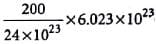= 50.19gmol−1 for fcc,  Z = 4, V = a3 =(200×10−10)3
d =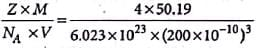= 41.66g cm3

Test: Calculations Involving Unit Cell Dimensions (NCERT) - Question 2

### A unit cell of sodium chloride has four formula units. The edge length of the unit cell is 0.564 nm. What is the density of sodium chloride?

Detailed Solution for Test: Calculations Involving Unit Cell Dimensions (NCERT) - Question 2

d =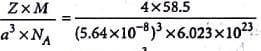= 2.16 gcm−3

Test: Calculations Involving Unit Cell Dimensions (NCERT) - Question 3

### The distance between Na+ and CI- ions in NaCl with a density 3.165 g cm-3 is

Detailed Solution for Test: Calculations Involving Unit Cell Dimensions (NCERT) - Question 3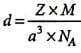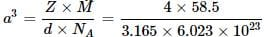a3 = 122.77 × 10−24cm3
a = 4.97 × 10−8 cm or 497 pm
Distance between Na+ and Cl = a/2
= 497/2
= 248.5 pm

Test: Calculations Involving Unit Cell Dimensions (NCERT) - Question 4

The unit cell of aluminium is a cube with an edge length of 405 pm. The density of aluminium is 2.70 g cm-3. What is the structure of unit cell of aluminium?

Detailed Solution for Test: Calculations Involving Unit Cell Dimensions (NCERT) - Question 4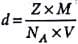⇒ 2.70 =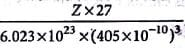Z = 4
Hence, structure of aluminium unit cell is fcc or face- centred.

Test: Calculations Involving Unit Cell Dimensions (NCERT) - Question 5

The density of a metal which crystallises in bcc lattice with unit cell edge length 300 pm and molar mass 50 g mol-1 will be

Detailed Solution for Test: Calculations Involving Unit Cell Dimensions (NCERT) - Question 5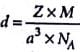For bcc structure, Z = 2
d =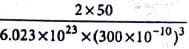= 6.15 g cm-3

## Chemistry Class 12

154 videos|396 docs|261 tests
Information about Test: Calculations Involving Unit Cell Dimensions (NCERT) Page
In this test you can find the Exam questions for Test: Calculations Involving Unit Cell Dimensions (NCERT) solved & explained in the simplest way possible. Besides giving Questions and answers for Test: Calculations Involving Unit Cell Dimensions (NCERT), EduRev gives you an ample number of Online tests for practice

## Chemistry Class 12

154 videos|396 docs|261 tests

### How to Prepare for NEET

Read our guide to prepare for NEET which is created by Toppers & the best Teachers(Scan QR code)